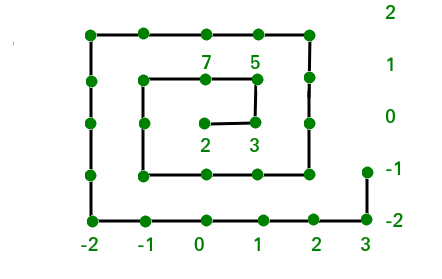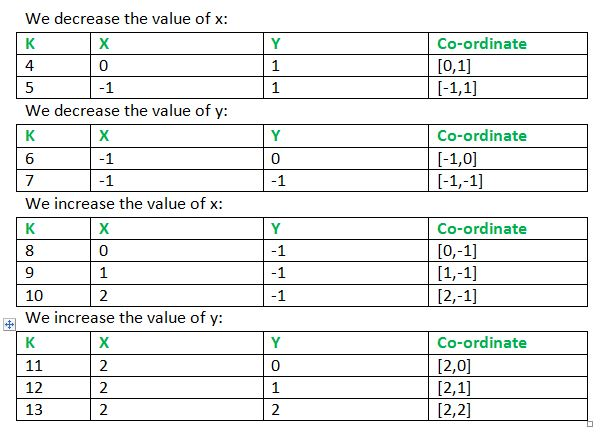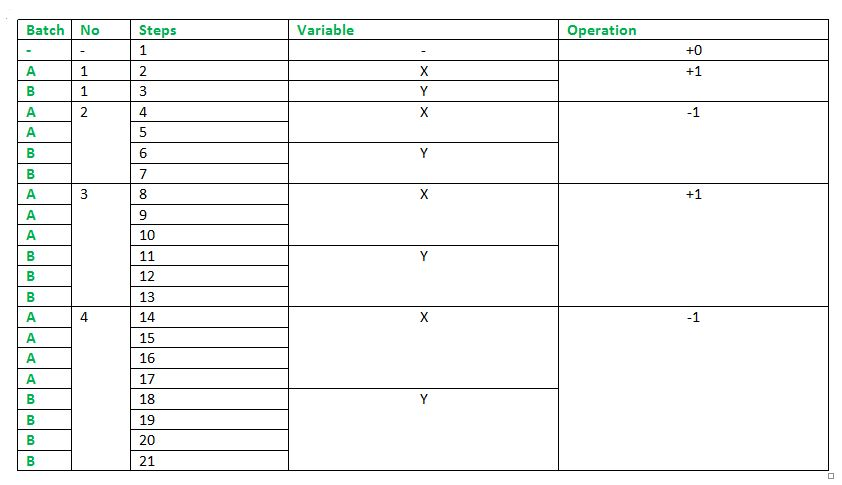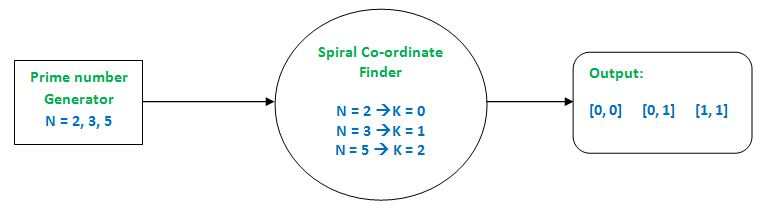# Find coordinates of a prime number in a Prime Spiral

Introduction
The Ulam spiral is a graphical depiction of the set of prime numbers, devised by mathematician Stanislaw Ulam. It is constructed by writing the positive integers in a square spiral and specially marking the prime numbers.You can read more about it here.

But we are going to compute on the alternate version of this spiral where the prime numbers are lined up in the spiral rather than natural numbers as in the original Ulam’s spiral.The prime numbers are written in a spiral form starting from the origin (0, 0) and moving as shown in the diagram above. The numbers shown in the right column and the bottom row are the column numbers and row numbers respectively ( i.e. y and x coordinates)

The objective is to find the position (x and y coordinates) of a given prime number.

Examples:

```Input : 5
Output : 1 1
As per the diagram above 5 corresponds to
the column 1 and row 1.

Input : 11
Output : -1 1
Similarly, 11 will correspond to column -1
and row 1.
```

## Recommended: Please try your approach on {IDE} first, before moving on to the solution.

Approach: The general solution to the problem would be to create an algorithm to predict the position of the moving spiral point at every step and reference each step in the spiral to a prime number (e.g. step 0 –> 2; step 1 –> 3 ; step 2 –> 5 and so on). Using this prime number we can trace back to the co-ordinates which is our solution (2 –> [0, 0], 3 –> [1, 0], 5 –> [1, 1]).

We begin by keeping count of x and y, for step k = 1 : x = y = 0. k = 2 leads to increase in x by 1 (x = 1) next comes k = 3 providing y = 1. Now as the spiral rotates we are to decrease the values now by 2. The consecutive movements are shown below:From the above computations, we observe that the operations are same for a batch of steps, e.g. at first K = 2 & 3 follow one where x is increased by 1 and then y by 1. Next in K= 4, 5, 6, 7 for 4 and 5, x is decreased and y is decreased in K = 6 and 7. Again From K = 8, 9 and 10, x is increased in repeated steps and 11, 12, 13 consists of decreasing y.

Each step can be classified into a batch and the size of batch increases by 1 after two runs. After a run, the operation is switched from y to x or vice versa and after every two batch runs the addition operation is switched to subtraction and vice versa. The following table explains it.Lastly, we also need to create a prime number generator that will keep on feeding the above algorithm with a prime number that will be referenced to a corresponding step and it will give us the required coordinates.Here is the implementation of above idea :
Input Limits : 2 < N < 1000000
Assumption : Prime numbers are the only inputs to the program.

## C++

 `// C++ Program to find coordinates of a ` `// prime number in a Prime Spiral ` `#include ` ` `  `using` `namespace` `std; ` ` `  `// The main algorithm that keeps track of ` `// coordinates ` `void` `spiralSplicer(``int` `input) ` `{ ` `    ``// Batch size tracker ` `    ``int` `step_count = 1; ` ` `  `    ``// Batch size limiter ` `    ``int` `step_limit = 2; ` ` `  `    ``// switches between -1 and +1 ` `    ``int` `adder = 1; ` ` `  `    ``// Co-ordinates of step K ` `    ``int` `x = 0, y = 0; ` ` `  `    ``for` `(``int` `n = 2; n != input + 1; n++,  ` `                       ``step_count++) {  ` ` `  `        ``// Keeps track of steps ` `        ``// Checks on the current batch ` `        ``if` `(step_count <= .5 * step_limit) ` `            ``x += adder; ``// Switch to operating on x ` ` `  `        ``else` `if` `(step_count <= step_limit) ` `            ``y += adder; ``// Switch to operating on x ` `         `  `        ``if` `(step_count == step_limit) { ` `  `  `            ``// Changes adder to -1 and vice versa ` `            ``adder *= -1;  ` ` `  `           ``// Keeps on updating 'step_limit' ` `           ``step_limit += 2;  ` `            `  `           ``// Resets count ` `           ``step_count = 0;  ` `        ``} ` `    ``} ` `    ``cout << x << ``" "` `<< y; ` `} ` ` `  `int` `primeIndex(``int` `input) ` `{ ` `    ``int` `j, cnt, prime_c = 0; ` `    ``for` `(``int` `i = 2; i <= 1000000; i++) { ` `        ``cnt = 0; ` `        ``for` `(j = 2; j <= i; j++) { ` `            ``if` `(i % j == 0) ` `                ``cnt++; ` `        ``} ` ` `  `        ``if` `(cnt == 1) { ` `            ``prime_c++; ` ` `  `            ``if` `(input == i) { ` ` `  `           ``/* Replaces the prime number with  ` `              ``Step K which will be fed into ` `              ``the main algorithm*/` `                ``input = prime_c;  ` `                ``break``; ` `            ``} ` `        ``} ` `    ``} ` `    ``return` `input; ` `} ` ` `  `// driver code ` `int` `main() ` `{ ` `    ``int` `input = 113; ` ` `  `    ``// Prime Index Finder Output ported ` `    ``// to final algorithm ` `    ``spiralSplicer(primeIndex(input)); ` `} `

## Java

 `// java Program to find coordinates of a ` `// prime number in a Prime Spiral ` `public` `class` `GFG { ` `     `  `    ``// The main algorithm that keeps track of ` `    ``// coordinates ` `    ``static` `void` `spiralSplicer(``int` `input) ` `    ``{ ` `         `  `        ``// Batch size tracker ` `        ``int` `step_count = ``1``; ` `     `  `        ``// Batch size limiter ` `        ``int` `step_limit = ``2``; ` `     `  `        ``// switches between -1 and +1 ` `        ``int` `adder = ``1``; ` `     `  `        ``// Co-ordinates of step K ` `        ``int` `x = ``0``, y = ``0``; ` `     `  `        ``for` `(``int` `n = ``2``; n != input + ``1``;  ` `                    ``n++, step_count++) ` `        ``{  ` `     `  `            ``// Keeps track of steps ` `            ``// Checks on the current batch ` `            ``if` `(step_count <= .``5` `* step_limit) ` `             `  `                ``// Switch to operating on x ` `                ``x += adder; ` `     `  `            ``else` `if` `(step_count <= step_limit) ` `             `  `                ``// Switch to operating on x ` `                ``y += adder; ` `             `  `            ``if` `(step_count == step_limit) ` `            ``{ ` `     `  `                ``// Changes adder to -1 and ` `                ``// vice versa ` `                ``adder *= -``1``;  ` `     `  `                ``// Keeps on updating 'step_limit' ` `                ``step_limit += ``2``;  ` `                     `  `                ``// Resets count ` `                ``step_count = ``0``;  ` `            ``} ` `        ``} ` `         `  `        ``System.out.print( x + ``" "` `+ y); ` `    ``} ` `     `  `    ``static` `int` `primeIndex(``int` `input) ` `    ``{ ` `        ``int` `j, cnt, prime_c = ``0``; ` `        ``for` `(``int` `i = ``2``; i <= ``1000000``; i++)  ` `        ``{ ` `            ``cnt = ``0``; ` `            ``for` `(j = ``2``; j <= i; j++) ` `            ``{ ` `                ``if` `(i % j == ``0``) ` `                    ``cnt++; ` `            ``} ` `     `  `            ``if` `(cnt == ``1``)  ` `            ``{ ` `                ``prime_c++; ` `     `  `                ``if` `(input == i)  ` `                ``{ ` `     `  `                    ``/* Replaces the prime  ` `                    ``number with Step K which  ` `                    ``will be fed into ` `                    ``the main algorithm*/` `                    ``input = prime_c;  ` `                    ``break``; ` `                ``} ` `            ``} ` `        ``} ` `         `  `        ``return` `input; ` `    ``}      ` `     `  `    ``// Driver code ` `    ``public` `static` `void` `main(String args[]) { ` ` `  `        ``int` `input = ``113``; ` ` `  `        ``// Prime Index Finder Output ported ` `        ``// to final algorithm ` `        ``spiralSplicer(primeIndex(input)); ` ` `  `    ``} ` `} ` ` `  `// This code is contributed by Sam007. `

## Python

 `# Python Program to find coordinates of a ` `# prime number in a Prime Spiral ` ` `  `# The main algorithm that keeps track of ` `# coordinates ` `def` `spiralSplicer(inp): ` `    `  `    ``# Batch size tracker ` `    ``step_count ``=` `1` `  `  `    ``# Batch size limiter ` `    ``step_limit ``=` `2` `  `  `    ``# switches between -1 and +1 ` `    ``adder ``=` `1` `  `  `    ``# Co-ordinates of step K ` `    ``x, y ``=` `0``, ``0` `  `  `    ``for` `n ``in` `range``(``2``, inp ``+` `1``): ` `    `  `        ``# Keeps track of steps ` `        ``# Checks on the current batch ` `        ``if` `(step_count <``=` `.``5` `*` `step_limit): ` `            ``x ``+``=` `adder ``# Switch to operating on x ` `  `  `        ``elif` `(step_count <``=` `step_limit): ` `            ``y ``+``=` `adder ``# Switch to operating on x ` `          `  `        ``if` `(step_count ``=``=` `step_limit): ` `            ``# Changes adder to -1 and vice versa ` `            ``adder ``*``=` `-``1` `  `  `            ``# Keeps on updating 'step_limit' ` `            ``step_limit ``+``=` `2` `             `  `            ``# Resets count ` `            ``step_count ``=` `0` `        ``step_count ``+``=` `1` `    ``print` `x, y ` `  `  `def` `primeIndex(inp): ` `    ``cnt, prime_c ``=` `0``, ``0` `    ``for` `i ``in` `range``(``2``, ``1000000` `+` `1``): ` `        ``cnt ``=` `0` `        ``for` `j ``in` `range``(``2``, i ``+` `1``): ` `            ``if` `(i ``%` `j ``=``=` `0``): ` `                ``cnt ``+``=` `1` `         `  `        ``if` `(cnt ``=``=` `1``): ` `            ``prime_c ``+``=` `1` `  `  `            ``if` `(inp ``=``=` `i): ` `  `  `                ``""" Replaces the prime number with  ` `                    ``Step K which will be fed into ` `                    ``the main algorithm """` `                ``inp ``=` `prime_c ` `                ``break` `    ``return` `inp ` `  `  `# driver code ` `inp ``=` `113` ` `  `# Prime Index Finder Output ported ` `# to final algorithm ` `temp ``=` `primeIndex(inp) ` ` `  `spiralSplicer(temp) ` ` `  `#This code is contributed by Sachin Bisht `

## C#

 `// C# Program to find coordinates of a ` `// prime number in a Prime Spiral ` `using` `System; ` ` `  `class` `GFG { ` `     `  `    ``// The main algorithm that keeps track of ` `    ``// coordinates ` `    ``static` `void` `spiralSplicer(``int` `input) ` `    ``{ ` `         `  `        ``// Batch size tracker ` `        ``int` `step_count = 1; ` `     `  `        ``// Batch size limiter ` `        ``int` `step_limit = 2; ` `     `  `        ``// switches between -1 and +1 ` `        ``int` `adder = 1; ` `     `  `        ``// Co-ordinates of step K ` `        ``int` `x = 0, y = 0; ` `     `  `        ``for` `(``int` `n = 2; n != input + 1;  ` `                     ``n++, step_count++) ` `        ``{  ` `     `  `            ``// Keeps track of steps ` `            ``// Checks on the current batch ` `            ``if` `(step_count <= .5 * step_limit) ` `             `  `                ``// Switch to operating on x ` `                ``x += adder; ` `     `  `            ``else` `if` `(step_count <= step_limit) ` `             `  `                ``// Switch to operating on x ` `                ``y += adder; ` `             `  `            ``if` `(step_count == step_limit) ` `            ``{ ` `     `  `                ``// Changes adder to -1 and ` `                ``// vice versa ` `                ``adder *= -1;  ` `     `  `                ``// Keeps on updating 'step_limit' ` `                ``step_limit += 2;  ` `                     `  `                ``// Resets count ` `                ``step_count = 0;  ` `            ``} ` `        ``} ` `         `  `        ``Console.Write( x + ``" "` `+ y); ` `    ``} ` `     `  `    ``static` `int` `primeIndex(``int` `input) ` `    ``{ ` `        ``int` `j, cnt, prime_c = 0; ` `        ``for` `(``int` `i = 2; i <= 1000000; i++)  ` `        ``{ ` `            ``cnt = 0; ` `            ``for` `(j = 2; j <= i; j++) ` `            ``{ ` `                ``if` `(i % j == 0) ` `                    ``cnt++; ` `            ``} ` `     `  `            ``if` `(cnt == 1)  ` `            ``{ ` `                ``prime_c++; ` `     `  `                ``if` `(input == i)  ` `                ``{ ` `     `  `                    ``/* Replaces the prime  ` `                    ``number with Step K which  ` `                    ``will be fed into ` `                    ``the main algorithm*/` `                    ``input = prime_c;  ` `                    ``break``; ` `                ``} ` `            ``} ` `        ``} ` `         `  `        ``return` `input; ` `    ``}      ` `     `  `    ``// Driver code ` `    ``public` `static` `void` `Main () ` `    ``{ ` `        ``int` `input = 113; ` ` `  `        ``// Prime Index Finder Output ported ` `        ``// to final algorithm ` `        ``spiralSplicer(primeIndex(input)); ` `    ``}         ` `} ` ` `  `// This code is contributed by Sam007. `

## PHP

 ` `

Output :

```3 2
```

This article is contributed by Pritom Gogoi. If you like GeeksforGeeks and would like to contribute, you can also write an article using contribute.geeksforgeeks.org or mail your article to contribute@geeksforgeeks.org. See your article appearing on the GeeksforGeeks main page and help other Geeks.

My Personal Notes arrow_drop_up

Improved By : Sam007, jit_t

Article Tags :
Practice Tags :

1

Please write to us at contribute@geeksforgeeks.org to report any issue with the above content.